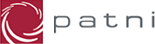# Placement Papers - PatniPATNI PAPER - 1999 - JADAVPUR
Posted by :
Sri
(6)
1. Number of Weights needed for weighing 1 to 40 kgs?
27 9 3 1 ans : 4
2. Venn diagram problem venndiagram find the
formulae.
Ans. (AB+BC+CA)(A\'+B\'+C\')
3. a+2b=6,ab=4 2/a + 1/b=?
ans : 3/2
4. age of 4 persons given in a relation find smallest age?
ans : meera
5. find floating point result
(float)25/2, float(25/2), 25/(float)2 ,25/2
ans: 1 & 3

6. find equivalent *(*(s+x)+y) == s[x][y]
ans : content of s[x][y]

7. find invalid statement : int a
1. scanf("%d",a);
2. scanf("%d",&a);
3. scanf("%d",a);
4. all

ans : 1

8.one palindrome programme was given in recursion
ans : pal(f++,t--)

9. main program:
i=foo(2) foo(int s)
{
if(!s)
return s;
else
{ int i=5; return i}
}
ans : 5

10.Ans.
data,execute,stroe

11.
k=0 i=0j=1;
if(i0)&&(k=2) printf(k);
if(i0|| k=0)
printf(k)

ans: 2 2

12.cube is moulded as a sphere. find the ratio of cube of surface area of sphere to squareof surface area of cube. ans pi/6

13. teacher in math=4 p6=3 kmistry=3 each can take 2 subject minimum teacher needed for school
ans : 5

14. 3 vowels,4 consonents formulate string of 6 letters with a vowel in a string max no of string ? ans: 5040

15.binnary of .6875.
0.1011

16. man walks at speed of 8 km/h 300 mtrs length train crosses man in 30
sec from back, find speed of train
ans: 44 km/hr

17. chess corner
ans : 30

18
input : " this is a line."

main()
{
char ch;
int count=0;
while((ch = getch() != \' \')
{
while (ch == \' \')
ch = getch();
while((ch!=\' \') && (ch!=\'.\'))
{
count++;
ch=getch();
}
}

ans : 11

19. radius of a circle is 5cm , if we draw a rectangle of maximum size, what is the area of rectangle
ans : 50 cmsq

20.
main()
{
int i;
for(i=0;i<3;i++)
switch(i)
{
case 1: printf("%d",i);
case 2 : printf("%d",i);
default: printf("%d"i);
}
}
ans 011122

Short listed have to appear for GD which includes filteration. GD :

1. Report to the commisioner about the Corporation Probs,
2. 5 things that u will consider while starting a company,
3. there is a ship u r given five persons and u have to send only three whom
will u send and why (Doctor, Engineer, lawer etc.),
4. what are the qulification required to have a high standards.
5.men are from mars and women are from venus.
6.what are the necessary steps should the senior management should take when the company has just launched and going to produce a software
product. at M.S. university
7. Should politician poses a minimum qulification.
8. Should India bid for organizing Olympic games.

This is the latest patni paper(campus recritment)
Basically there is a
1.apti(general+tech)
2.gd
3.pschyometric test
4.interview(tech+personal)

the apti consist of 20 questions time 50 min

5.there is a circle of radius 5.what is the area of the biggest rectangle that
can be cut out of this circle
6.in a chess board 2 squares are cut out leaving 61 squares if we place blocks
each of length=lengthof 2 squares and width=width of 1 square how many blocks will be necessary
9.radius =3 of a sphere it is melted to form a cube.what is the ratio of the
cube of the volume of the sphere to the cube of the volume of the cube

PCS PAPER (PATNI)
4. #include
/* This problem is given in PCS BOMBAY walk-in-interview.
* What is the final value of i and how many times loop is
* Executed ?
*/

main()

{
int i,j,k,l,lc=0;
/* the input is given as 1234 567 */
printf("Enter the number string:<1234 567
");
scanf("%2d%d%1d",&i,&j,&k);
for(;k;k--,i++)
for(l=0;l printf("%d %d
",i,l);}
printf("LOOPS= %d
", lc-1);
}
/* Ans: i = 17, and loop is executed for 169 times */
~

10.#include
main()
{
func(1);
}
func(int i){
static char *str[] ={ "One","Two","Three","Four"};
printf("%s
",str[i++]);
return;
}
/* Ans:- it will give warning because str is pointer to the char but
it is initialized with more values
if it is not considered then the answer is Two */

11.
#include
main()
{
int i;
for (i=1;i<100; i++)
printf("%d %0x
",i,i);
}
/* Ans:- i is from 1 to 99 for the first format,
for the second format 1to9, ato f, 10 to 19,1ato1f, 20 to 29, etc */

13.#include
/* This problem was asked in PCS Bombay in a walk-in-interview
* Write a recursive function that calculates
* n * (n-1) * (n-2) * ....... 2 * 1
*/

main() {
int factorial(int n);
int i,ans;
printf("
Enter a Number:");
scanf("%d",&i);
ans = factorial(i);
printf("
Factorial by recursion = %d
", ans);
}
int factorial(int n)
{
if (n <= 1) return (1);
else
return ( n * factorial(n-1));
}

14.#include
/* This problem is asked in PCS Bombay walk-in-interview
* What is the output of the following problem
*/
main(){
int j,ans;
j = 4;
ans = count(4);
printf("%d
",ans);
}
int count(int i)
{
if ( i < 0) return(i);
else
return( count(i-2) + count(i-1));
}

/* It is showing -18 as an answer */

4. #include
/* This problem is given in PCS BOMBAY walk-in-interview.
* What is the final value of i and how many times loop is
* Executed ?
*/

main()

{
int i,j,k,l,lc=0;
/* the input is given as 1234 567 */
printf("Enter the number string:<1234 567
");
scanf("%2d%d%1d",&i,&j,&k);
for(;k;k--,i++)
for(l=0;l printf("%d %d
",i,l);}
printf("LOOPS= %d
", lc-1);
}
/* Ans: i = 16, and loop is executed for 169 times */